# The audiometry package

### In Short

If you deal with something like this, then this package is for you:

exmpl <- data.frame(f = c(125,250,500,1000,2000,3000,4000,8000),
t = c(15,10,25,35,40,55,75,60))
library(audiometry)
library(ggplot2)
gg_pta(data.frame(exmpl)) +
geom_point(aes(x=f, y=t), color="red", size=2.5) +
geom_line(aes(x=f, y=t), color="red", lwd=.8)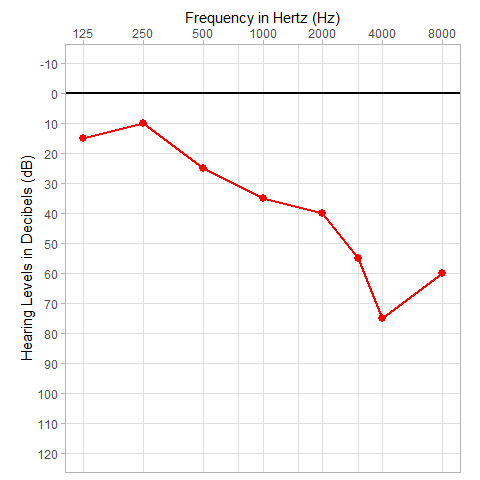### A bit longer

More then 30,000,000 people in the USA suffer from hearing loss, mostly the elderly, >80% at 85 years old or above. For more background and details on epidemiology see http://dx.doi.org/10.4236/ijohns.2013.24025 . Treatment is of utmost importance and great progress has been made in understanding, detecting as well as treating different conditions that lead to hearing loss, yet the situation is often dissatisfying and just a glance at the numbers of the affected, the huge impact on their lives and cost of some treatment options like cochlear implants serve as proof, that the topic deserves further research.

Among the many approaches to measure hearing loss, pure tone audiometry (PTA) clearly plays the most important role, up to the point, where laymen call pure tone audiometry just “hearing test”.

In the simplest form, a patient is given headphones, sinus tones in different frequencies are played at different intensities and hearing thresholds are marked in a standardized diagram. Results are usually communicated visually via this diagram and thus it is only natural, to use the same diagram standards in scientific publication of pure tone audiometric data.

### Standards to be met

There is an ISO standard ISO 8253-1:2010(en) that is not open, so the author could not refer to it. The American Speech-Languge-Hearing Association (ASHA) has published their guidelines openly ( https://www.asha.org/policy/GL2005-00014/#sec1.8 ) where they state:

When the graphic form is used, the test frequencies shall be recorded on the abscissa, indicating frequency on a logarithmic scale, and hearing levels shall be recorded on the ordinate, using a linear scale to include the units of decibels. The aspect ratio of the audiogram is important for standardization. The correct aspect ratio is realized when a square is formed between any given octave pair on the abscissa and any 20 dB increment on the ordinate. For conventional audiometry, the vertical scale is to be designated hearing level in decibels; the horizontal scale is to be labeled frequency in hertz. By convention, frequency is recorded in ascending order from left to right, and hearing level is recorded in ascending order from top to bottom, ranging from a minimum value of −10 dB to the maximum output limits of the audiometer (usually 110 or 120 dB HL).

Whilst regarding all these rules is obviously possible in ggplot2 it is not necessarily easy nor will every scientist in the field of hearing research always have these rules in mind.

That is, why the audiometry package provides an easy way to prepare a suitable ggplot object to start building diagrams on.

### How to start

First, we define some example data with no particular meaning except for showing the functionality.

ex1 <- data.frame(time = gl(3, 6),
f = rep(c(250, 500, 1000, 2000, 4000, 8000), 3),
t = c(60, 65, 40, 25, 10, 5,
15, 25, 20, 10, 5, 0,
5, 5, 10, 0, 0, 10))

When starting a ggplot2 graphic, you usually start by calling ggplot with a data.frame as in

library(ggplot2)
ggpl <- ggplot(ex1)

Instead we will start a pure-tone-audiogram-like plot with

library(ggplot2)
library(audiometry)
ggpl <- gg_pta(ex1)

This will prepare our form. In the next step we need to add geoms to put our data into the form. Now this is all done with standard ggplot2 commands. In our case, let’s assume we optained a 6 frequency pure tone audiogram of a patient with sudden sensoryneural hearing loss in the deep frequencies at three different points in time: onset, two weeks and four weeks later, when she had totally recovered. We call gg_pta as our basis, then we add points with geom_point and lines with geom_line where line type and color represent the three points in time:

gg_pta(ex1) +
geom_point(aes(x = f, y = t, color = time), size = 3, alpha=.5) +
geom_line(aes(x = f, y = t, color = time, lty = time), lwd=1)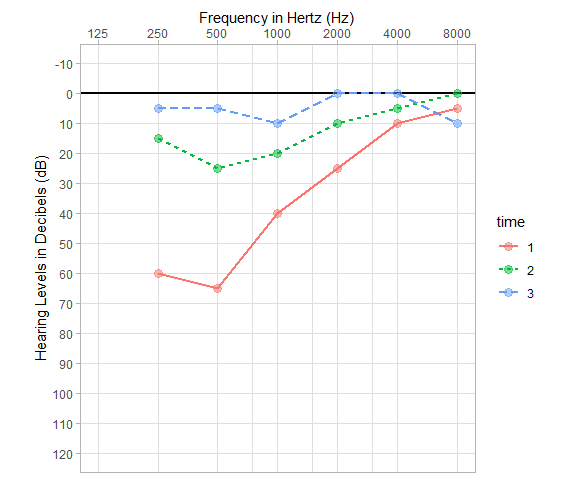### Making changes

There are two ways to make changes to this plot. Either through gg_pta itself or employing all the manyfold possbilities ggplot2 offers the adept. If you wanted to change the language of the axis labels and the axis limits and the extra line at 0 dB to disapear, that can easily be done when calling gg_pta:

gg_pta(data.frame(0), xlab = "frequenza [Hz]", ylab = "volume [dB]",
xlim = c(125, 12000), x_base_lwd = -1)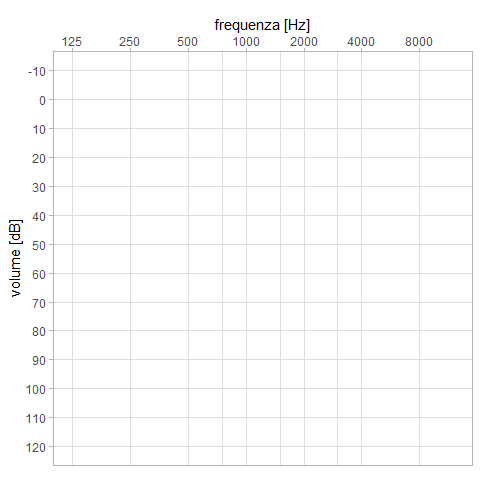All the possible arguments to gg_pta are in the manual, just type help(gg_pta)

### Marking sides

There is a number of ways to mark a side in PTA. gg_pta has a shortcut by means of the lettermark argument, which adds an “R” or “L” or both in size lettermarksize to the audiogram

gg_pta(data.frame(0), lettermark = c("R", "L"), lettermarksize = 40)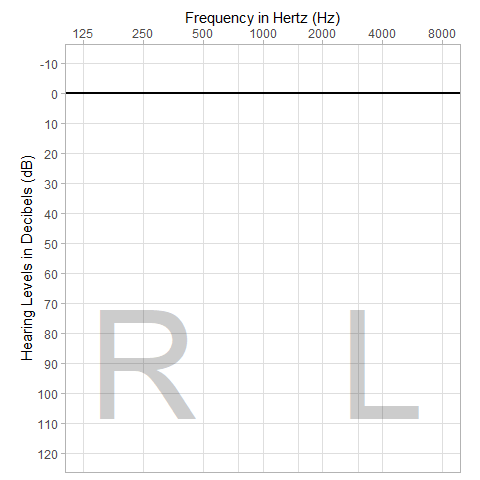### Examples

Obviously, for the ggplot2 savy, everything can be changed. Here are some examples with a randomly generated data set.

gg_pta(some.data) +
geom_line(aes(x = freq, y = thres, group = id, color = treatment)) +
theme_grey() +
theme(legend.position = c(0.5, 0.2))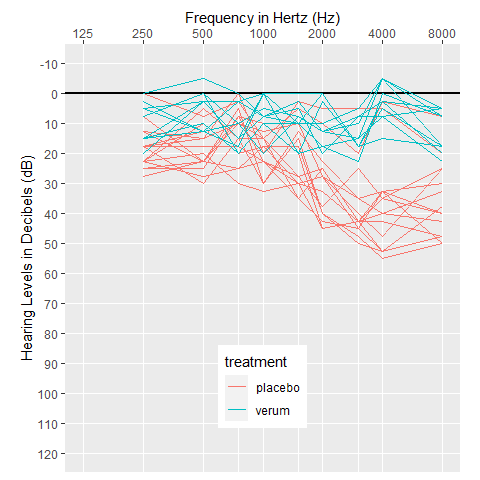library(ggbeeswarm)
gg_pta(some.data, xlim=c(125, 10000), x_base_lwd = 1.5,
xlab = "Frequenz [Hz]", ylab = "Lautstärkepegel [dB HL]") +
geom_beeswarm(aes(x = freq, y = thres, color=treatment), cex = 1.5) +
theme_linedraw()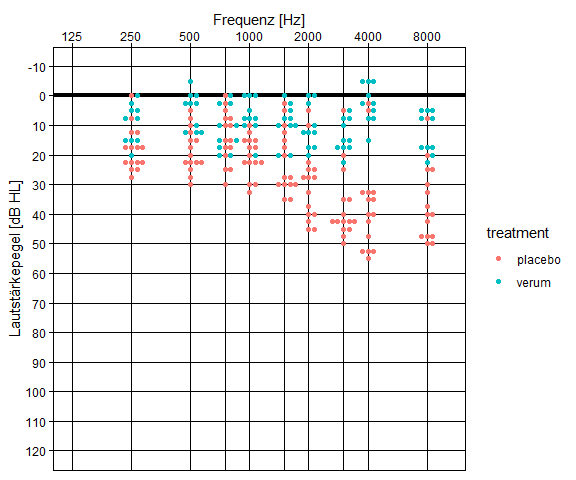In order to decide, whether insurances will pay for hearing aids, German ENT specialists have to check, whether at least two thresholds in between 0.5 kHz an 4 kHz are 30 dB or louder. That decision line is easily visualized:

library(ggthemes)
gg_pta(data.frame(0)) +
geom_segment(aes(x = 500, y = 29, xend = 4000, yend = 29), colour = "red", lwd=2) +
geom_segment(aes(x = 500, y = 31, xend = 4000, yend = 31), colour = "green", lwd=2) +
theme_gdocs()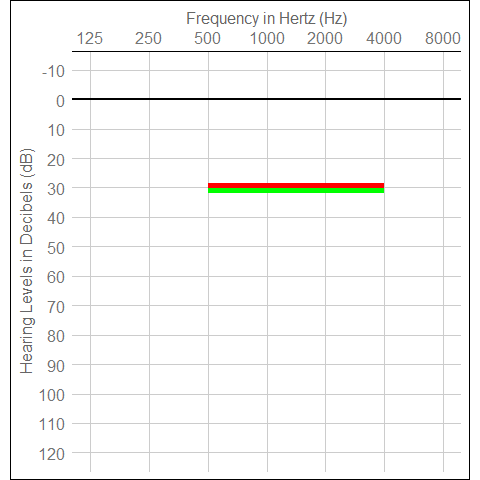### Finally

The package name audiometry is obviously much wider then just pure tone audiogram forms. I intend to broaden the package’s functionality slowly over time. As of now the audiometry packages has support for the Freiburger Sprachtest which is a widely used speech audiometry test in German speaking countries.

– Bernhard Lehnert